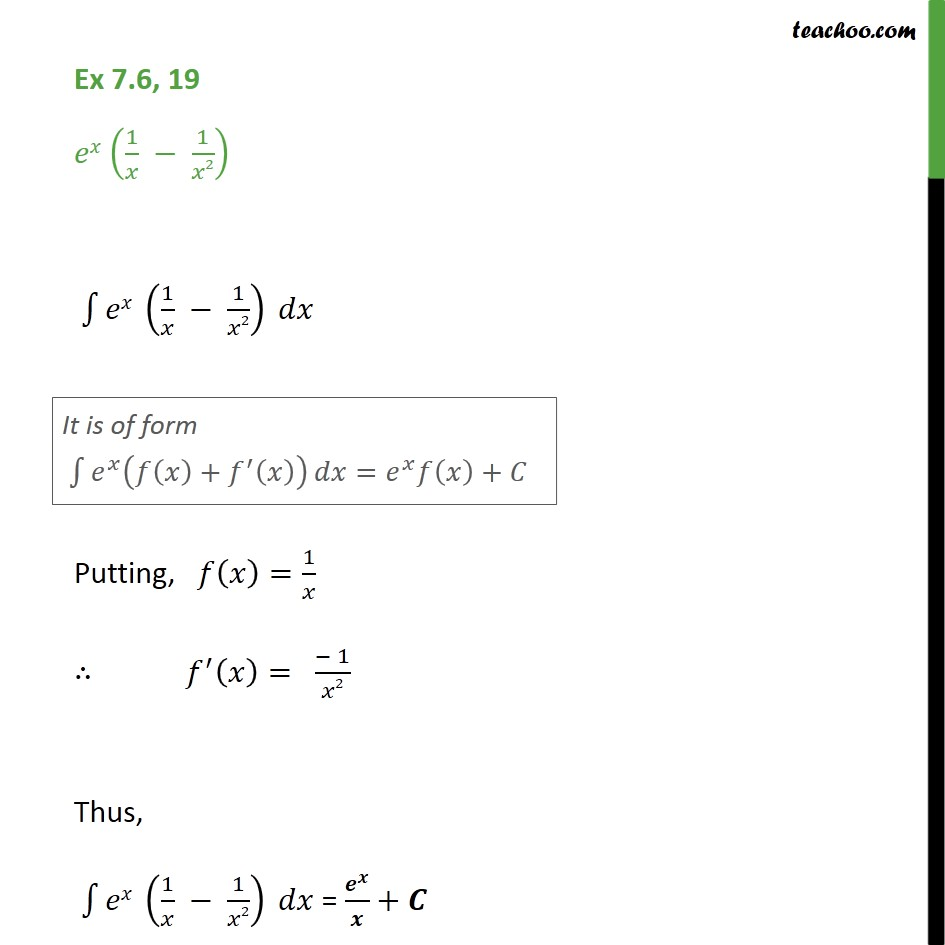1. Chapter 7 Class 12 Integrals (Term 2)
2. Concept wise
3. Integration by parts - e^x integration

Transcript

Ex 7.6, 19 - Chapter 7 Integration - Class 12 NCERT Book e^x (1/x - 1/x^2) ∫1▒〖𝑒𝑥" " (1/𝑥 − 1/𝑥2) 𝑑𝑥〗 It is of form ∫1▒〖𝑒^𝑥 (𝑓(𝑥)+𝑓^′ (𝑥)) 〗 𝑑𝑥=𝑒^𝑥 𝑓(𝑥)+𝐶 Putting, 𝑓(𝑥)=1/𝑥 " " ∴ 𝑓^′ (𝑥)= (− 1)/𝑥2 Thus, ∫1▒〖𝑒𝑥" " (1/𝑥 − 1/𝑥2) 𝑑𝑥〗 = 𝒆^𝒙/𝒙+𝑪

Integration by parts - e^x integration

Chapter 7 Class 12 Integrals (Term 2)
Concept wise